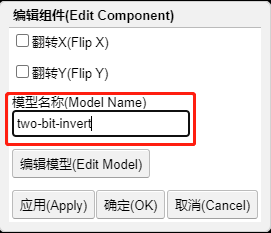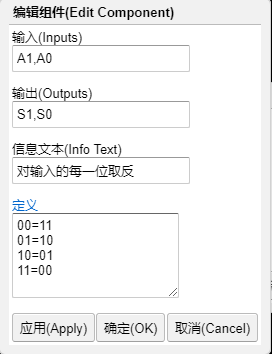Circuitjs 创建自定义逻辑(Custom Logic)器件

一个具体示例S1=!A1
S0=!A01. 输入

改为 A1,A0, 与原型一致, 以(英文)逗号分隔. 当然, 这里实际叫什么并不是特别重要, 根据你自己需要去定义即可, 但建议尽可能简短.

视具体情况不同, 输入端可以有更多或更少. 比如 X,Y,Z 就表示有三个输入端, 名称分别被设为 X, Y 和 Z.

2. 输出

改为 S1,S0, 与原型一致(同理, 这里实际叫什么也不是特别重要)

同样的, 输出端也不限于两个, 可以更多或更少.

3. 信息文本

这里可以填入对功能的一个具体描述, 比如"对输入的每一位取反"

4. 定义

这是最重要的, 它定义了输入输出间的逻辑关系.

00=11
01=10
10=01
11=00S1=!A1
S0=!A0

00=0
01=1
10=1
11=1111=0
???=1

111=11
110=10
011=10
101=10
100=01
010=01
001=01
000=00

?? 00=10
10 ??=10
01 ??=01
?? AB=AB

?? 00=10
0+ ??=01
1+ ??=10
?? AB=AB

??? 00=10
00- AB=AB
10- ??=10
01- ??=01
11- AB=BA
??? AB=AB

ABC ABC=100
1?? 0??=010
A1? A0?=010
AB1 AB0=010
??? ???=001

+ AB0=AB1
+ A01=A10
+ 011=100
+ 111=000
? ABC=ABC

1111=0
???1=1
???0=_

A1=A
?0=_